For the 1861 penny there are ten die varieties consisting of nine obverse dies paired with six reverse dies.  The differences can be noted in the date and in the fraction.

 1861Penny Obverse Reverse1861Penny Obverse Reverse1861Penny Obverse Reverse• Obverse 1S:
• The spacing between the one and the one is 5.98 mm.
• The spacing between the one and the eight is 1.11 mm.
• The spacing between the eight and the six is 0.79 mm.
• The spacing between the six and the one is 0.90 mm.
• The eight is below the horizontal while the six is slightly below the horizontal.
• The eight in the date has been significantly repunched.
• Striations are visible.
• Obverse 2:
• The spacing between the one and the one is 5.66 mm.
• The spacing between the one and the eight is 1.01 mm.
• The spacing between the eight and the six is 0.56 mm.
• The spacing between the six and the one is 0.95 mm.
• The eight and the six are on the horizontal.
• Obverse 2S:
• Similar to type 2 but with striations.

• Obverse 3:
• The spacing between the one and the one is 5.82 mm.
• The spacing between the one and the eight is 1.01 mm.
• The spacing between the eight and the six is 0.58 mm.
• The spacing between the six and the one is 1.06 mm.
• The the eight is slightly below the horizontal and the six is below the horizontal.
• Obverse 4S:
• The spacing between the one and the one is 6.83 mm.
• The spacing between the one and the eight is 1.01 mm.
• The spacing between the eight and the six is 0.58 mm.
• The spacing between the six and the one is 0.90 mm.
• The eight is below the horizontal and the six is on the horizontal.
• Striations are visible.
• Obverse 5:
• The spacing between the one and the one is 5.40 mm.
• The spacing between the one and the eight is 0.85 mm.
• The spacing between the eight and the six is 0.42 mm.
• The spacing between the six and the one is 0.74 mm.
• The eight and the six are on the horizontal.
• Obverse 6:
• The spacing between the one and the one is 5.29 mm.
• The spacing between the one and the eight is 0.90 mm.
• The spacing between the eight and the six is 0.53 mm.
• The spacing between the six and the one is 0.74 mm.
• The the eight is slightly below the horizontal and the six is slightly above the horizontal.
• Obverse 6S:
• Similar to type 6 but with striations.
• Obverse 7:
• The spacing between the one and the one is 5.50 mm.
• The spacing between the one and the eight is 0.95 mm.
• The spacing between the eight and the six is 0.51 mm.
• The spacing between the six and the one is 0.74 mm.
• The eight and the six are on the horizontal.
• Reverse A:
• The fraction has a rounded three.
• The second S in STATES has be repunched three times.
• Reverse B:
• The fraction with rounded three has been repaired.
• This is similar to the 1858 reverse die A.
• The second S in STATES has be repunched two times.
• Reverse C:
• The fraction has a sharp three.
• The E in STATES has be repunched.

• Reverse D:
• The fraction has a sharp three.
• The angle between the numerator and the bar is smaller when compared to the other dies.  The one of the denominator touches the bar.
• Reverse E:
• The fraction has a sharp three but the digits are small.
• The ES in STATES have not been repunched.
• Reverse F:
• The fraction has a sharp three but the one is larger than the three.The spacing is measured between the digits and the horizon is from the one to the one.

Obverse Dies:  The Date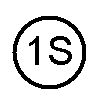Repunched 8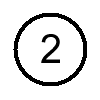Horizontal Date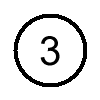Large spacing between the 6 and the 1Obverse Dies:  The Date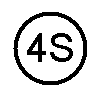Wide Date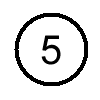Small Spacing between the 8 and the 6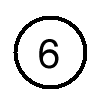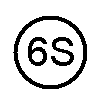Narrow DateObverse Dies:  The Date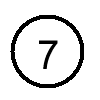Similar to type 5, but larger spacing between the 1 and the 8 and horizontal date.Obverse Dies:  The DateRepunched 8Horizontal DateLarge spacing between the 6 and the 1Wide DateSmall Spacing between the 8 and the 6Narrow DateSimilar to type 5,
but larger spacing between the 1 and the 8
and horizontal date.Reverse Dies:  1/13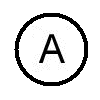Normal fractionRepaired fractionReverse Dies:  1/13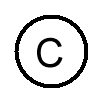Short fractionShort fraction with small angleReverse Dies:  1/13Fraction with sharp 3 and shorter digits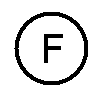Fraction with a small 3Reverse Dies:  TES of STATESThe repunched SThe repunched SThe repunched EReverse Dies:  TES of STATESNo repunched lettersReverse Dies:  1/13Normal fractionRepaired fractionShort fractionShort fraction with small angleFraction with sharp 3 and shorter digitsFraction with a small 3Reverse Dies:  TES of STATESThe repunched SThe repunched SThe repunched ENo repunched letters